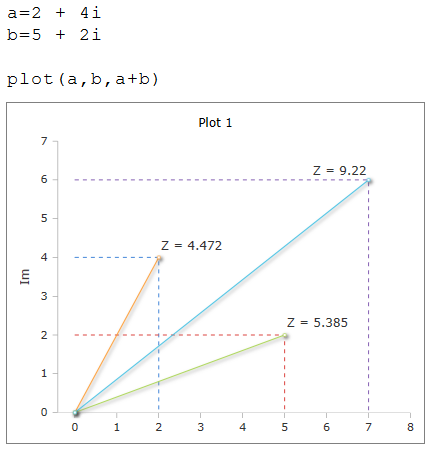# Plot Function

Visualize of complex numbers

### Description

The function $$Plot$$ visualizes complex numbers.

A complex number can be represented by its coordinates (polar coordinates) on a complex plane. The Plot function can represent single or multiple complex numbers separated by commas.

To execute the function, a plot box must be open.

### Syntax

Plot (complex)

Plot (complex, complex, ...complex)

### Example